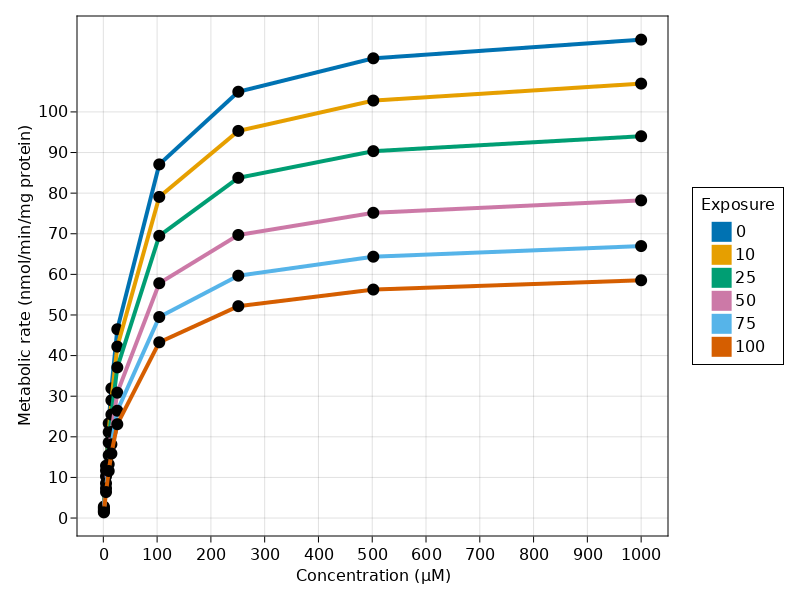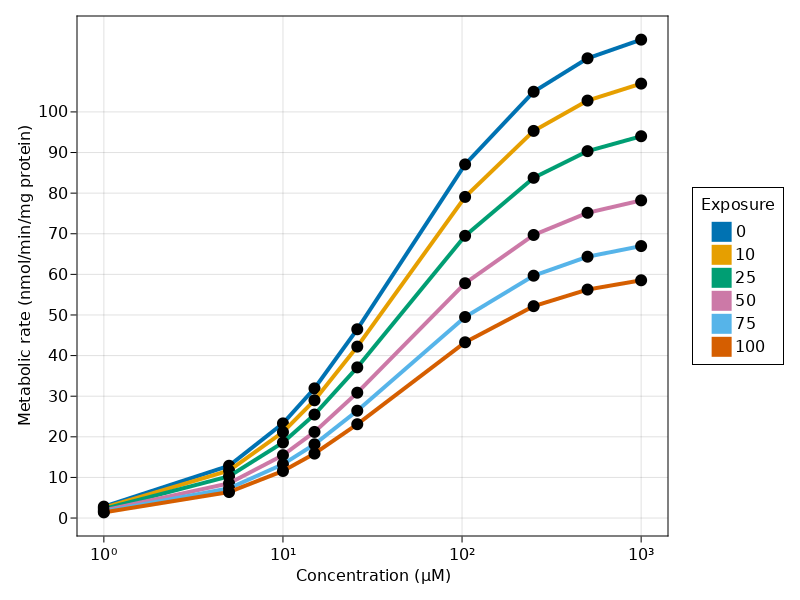# Exercise PK44_2 - Estimation of inhibitory constant Ki

### Background

• Structural model - Estimation of inhibitory rate constant in non-competitive enzyme inhibition model

• Number of subjects - 1

• Number of compounds - 1

### Learning Outcome

• To get an understanding about inhibitory models

• To understand the relationship between rate of metabolite formation and concentration

### Objectives

• To analyze enzyme data by means of simultaneous nonlinear regression

### Libraries

call the necessary 'libraries' to get started

using Random
using Pumas
using PumasUtilities
using CairoMakie
using AlgebraOfGraphics
using DataFramesMeta


### Model - Non-Competitive Inhibitory Model

pk_44_nim  = @model begin
desc         = "Competitive Inhibitory Model"
timeu        = u"minute"
end

@param begin
"Maximum metabolic rate (μM*gm/min)"
tvvmax       ∈ RealDomain(lower=0)
"Michaelis-Mentons constant (μmol/L)"
tvkm         ∈ RealDomain(lower=0)
"Inhibitory constant (μmol/L)"
tvki         ∈ RealDomain(lower=0)
Ω            ∈ PDiagDomain(2)
end

@random begin
η      ~ MvNormal(Ω)
end

@covariates conc I

@pre begin
Vmax   = tvvmax * exp(η)
Km     = tvkm * exp(η)
Ki     = tvki
_conc  = conc
_I     = I
end

@derived begin
## Noncompetitive Inhibition Model
rate_nim     = @. ((Vmax * _conc/(Km + _conc)) * (Ki / (Ki + _I)))
"""
Metabolic rate (nmol/min/mg)
"""
end
end

PumasModel
Parameters: tvvmax, tvkm, tvki, Ω, σ_add
Random effects: η
Covariates: conc, I
Dynamical variables:
Derived: rate_nim, dv_rate_nim
Observed: rate_nim, dv_rate_nim


### Parameters

Parameters provided for simulation are as below.tv represents the typical value for parameters.

• $Vmax$ - Maximum metabolic rate (μM*gm_protein/min)

• $Km$ - Michaelis-Mentons constant (μmol/L)

• $Ki$ - Inhibitory constant (μmol/L)

• $I$ - Inhibitor concentration/Exposure

param = ( tvvmax = 122.827,
tvkm   = 42.7053,
tvki   = 98.8058,
Ω      = Diagonal([0.0,0.0]),

(tvvmax = 122.827, tvkm = 42.7053, tvki = 98.8058, Ω = [0.0 0.0; 0.0 0.0],


### Creating a Dataset

In this dataset we will be having time, concentration data and Exposure(I) which will be used to estimate the rate of metabolite concentration

df_sub1 = map(i -> DataFrame(id=i,time = 1:1:1000, dv_rate_nim = missing, conc = 1:1:1000, I=0), 1:6)
df      = vcat(DataFrame.(df_sub1)...)
df[!, :I] = ifelse.(df.id .== 2, 10, df.I)
df[!, :I] = ifelse.(df.id .== 3, 25, df.I)
df[!, :I] = ifelse.(df.id .== 4, 50, df.I)
df[!, :I] = ifelse.(df.id .== 5, 75, df.I)
df[!, :I] = ifelse.(df.id .== 6, 100, df.I)
df_sub1 = df
sub1    = read_pumas(df_sub1, observations=[:dv_rate_nim], covariates=[:conc, :I], event_data=false)

Population
Subjects: 6
Covariates: conc, I
Observations: dv_rate_nim


### Simulation

We will now simulate the rate of metabolite formation

Random.seed!(123)
sim_sub1 = simobs(pk_44_nim, sub1, param)
df44_2      = DataFrame(sim_sub1)


### Visualization

##### Non-Competitive Inhibitory Model
@chain df44_2 begin
@rsubset :conc ∈ [1,5,10,15,26,104,251,502,1000]
data(_) *
mapping(:conc => "Concentration (μM)",
:rate_nim => "Metabolic rate (nmol/min/mg protein)",
color = :I => nonnumeric => "Exposure") *
visual(ScatterLines, linewidth = 4, markersize = 12)
draw(axis = (;xticks = 0:100:1000, yticks = 0:10:100))
end@chain df44_2 begin
@rsubset :conc ∈ [1,5,10,15,26,104,251,502,1000]
data(_) *
mapping(:conc => "Concentration (μM)",
:rate_nim => "Metabolic rate (nmol/min/mg protein)",
color = :I => nonnumeric => "Exposure") *
visual(ScatterLines, linewidth = 4, markersize = 12)
draw(axis = (;xscale = log10, yticks = 0:10:100))
end